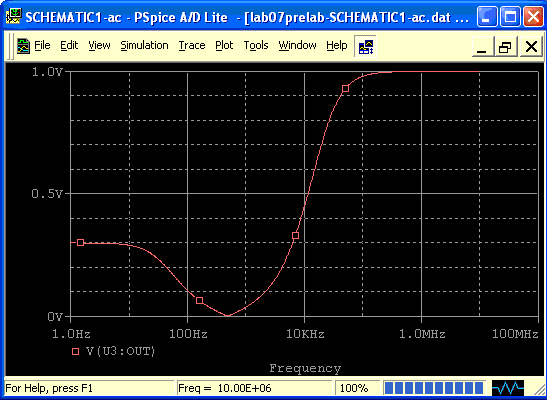Bill Chun Wai Hung

Lab 7 Prelab

Q1) Compute the cutoff frequency of the low pass filter of figure 1 considering it disconnected form the amplifier.

Low Pass Filter

R2 = 4k ohm

C2 = 1u ohm

f2 = 40 Hz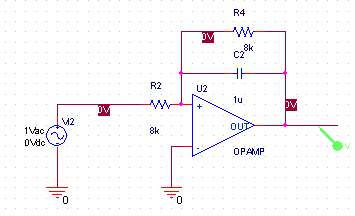Result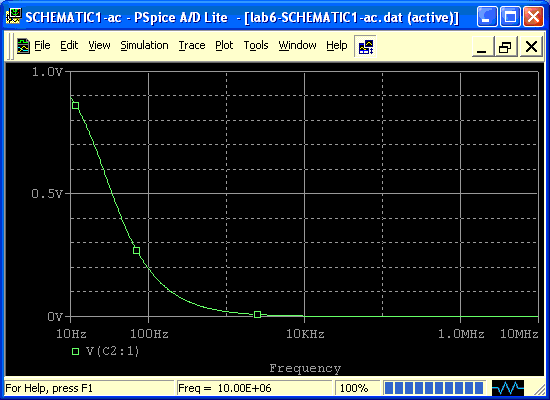High Pass Filter

R1 = 8k ohm

C1 = 1n ohm

f1 = 20kHz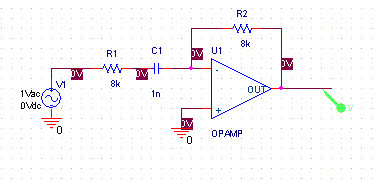Result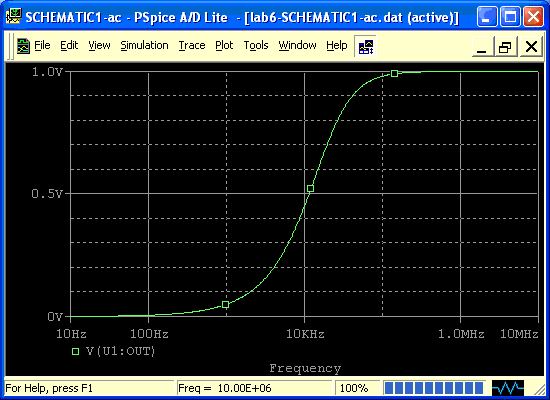Q2) Compute the cutoff frequency of the low pass filter of figure 1 When it is connected to the amplifier.

Low Pass

First calculate the Thevenin Resistance of the Amplifier

R(TH) =Vt/It

-Vt/RL ¨C Vo/R3=0

Vt = -Vo* RL/R3

It = -Vo/R3

R(TH) =Vt/It = RL

High Pass

-Vt/RH ¨C Vo/R3=0

Vt = -Vo* RH/R3

It = -Vo/R3

R(TH) =Vt/It = RH

Q3) The cutoff frequency of the input resistance of the amplifier should be very small in order to have the cutoff frequency to remain unchanged. Because the gain of the amplifier = -R3/ RL

So Ra should be very large.

Q4) The high pass filter data is shown above.

Q5)

R3/Ra=1

R3=Ra

R3/(Ra+10k) = 0.3

R3/(R3+10k) = 0.3

0.7 R3 = 3k

R3 = 4.29k

Ra = 4.29k

R3 = 4.29k

R1= 8k

C1 = 1n

R2 = 4k

C2 = 1u

The Circuit DiagramThe Result Plot